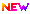High Impact Factor : 4.396| Submit Manuscript Online|

## Parameter Sensitivity Analysis of Cardiac Purkinje Fiber Single Cell Model Using Partial Least Square Regression

### Author(s):

Durga Choudhary , M.B.M Engineering College, J.N.V.U, Jodhpur; Dr. Akhil Ranjan Garg, M.B.M Engineering College, J.N.V.U, Jodhpur

### Keywords:

ionic conductance, injected current, regression coefficient, residual, standard deviation

### Abstract

In traditional method of parameter sensitivity analysis only one variable can be varied, and then impact on the output is observed, but the correlation between input variables and its effect on output was not observed. To overcome this problem the new technique was developed by Wold et al. In this technique we can analyze or predict a set of dependent variables from a set of independent variables (predictors). This prediction is done by a set of orthogonal factors (latent variables) obtained from a set of predictors and these latent variables have better predictive power. It combines the features of PCA and multiple linear regression. The PLS regression technique is used to determine the strength of the impact of multiple independent variables on a dependent variable using regression coefficient matrix which is a reflection of parameter sensitivities of input parameters. In this work we have done parameter sensitivity analysis of the already existing cardiac single cell Purkinje fiber (Noble) model.

### Other Details

Paper ID: IJSRDV7I120002
Published in: Volume : 7, Issue : 12
Publication Date: 01/03/2020
Page(s): 59-62